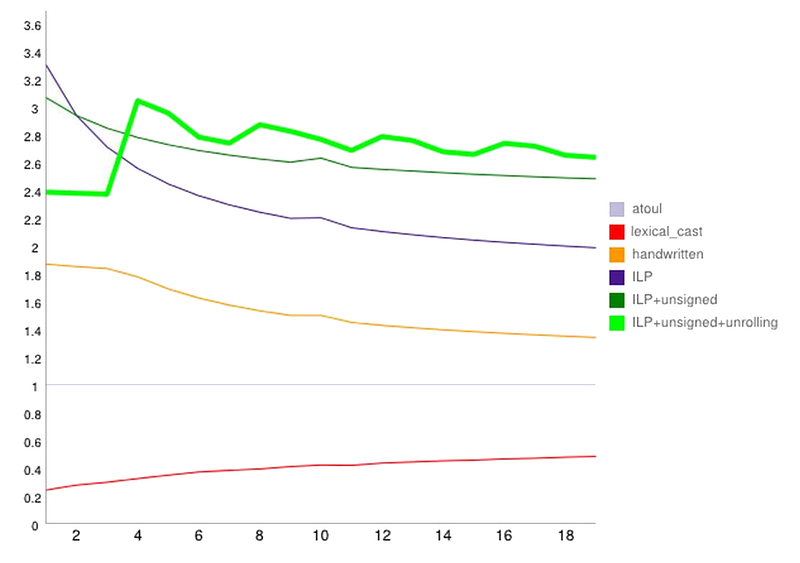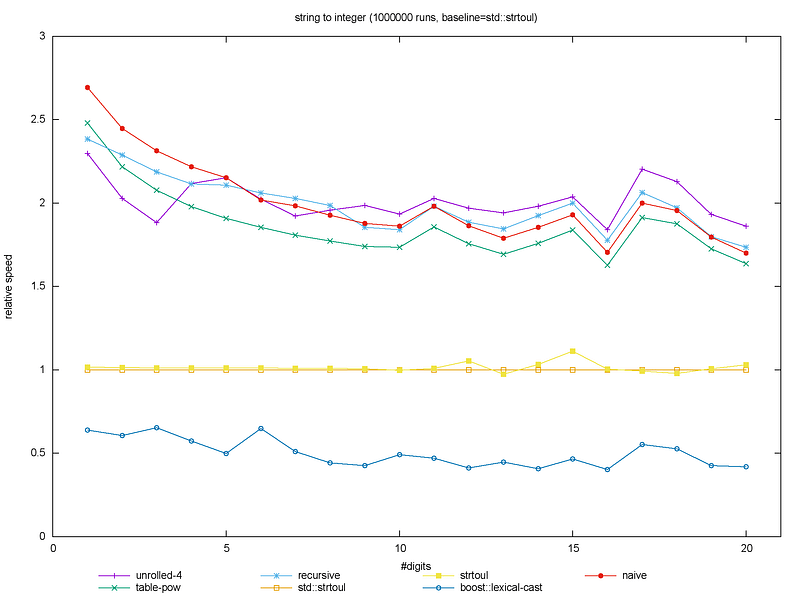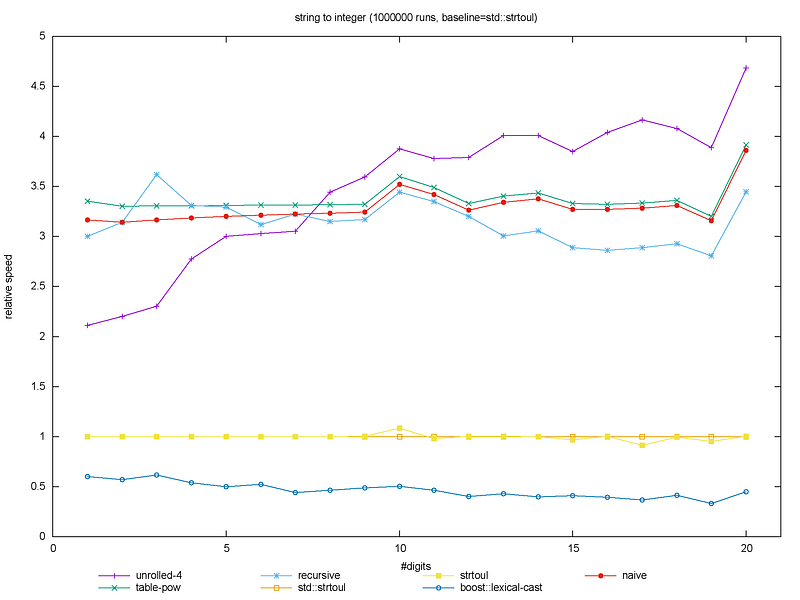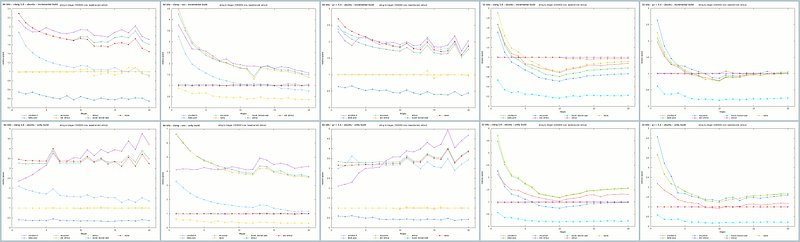Julien Jorge's Personal Website

## Benchmarking atoui(): a follow-up to Writing Fast Code

Wed Sep 21, 2016

I have been watching the excellent talk “Writing Fast Code” by Andrei Alexandrescu with some colleagues recently. In this talk the speaker focuses on the low level optimizations and performance improvements of C++ code while emphasizing on the importance of measuring. This is a very pleasant technical talk.

An astonishing point in the talk concerns the benchmarks. The author takes some textbook case algorithms and implement them using some nice optimization techniques such that they outperform the version of the standard library. By the end, I felt the need to see the results by myself. Here are my experiments.

First of all, if you have not watched the talk yet, I strongly encourage you to do so (even if it is not necessary to understand this article): here are the first part and the second part.

# Avoiding data dependency

Three times in the talk Alexandrescu presents a classic problem and a naive algorithm to solve it. Each time, he progressively lists the flaws in the implementation, then describes a more efficient way to do the job, and finally he compares his implementations with both the naive version and the one available in the standard library.

One of these problem is the conversion of a number from a string representation to an integer. You may recognize here a function such as `atoui()` or `strtoul()`. A naive implementation of this algorithm is

``````std::uint64_t atoi( const char* first, const char* last )
{
std::uint64_t result( 0 );

for ( ; first != last; ++first )
{
enforce( ( '0' <= *first ) && ( *first <= '9' ) );
result = result * 10 + ( *first - '0' );
}

return result;
}
``````

Where enforce( bool ) could be implemented like

``````void enforce( bool b )
{
if ( !b )
throw some_exception();
}
``````

As Alexandrescu tells us, the main problem in this implementation is the data dependency which prevents the CPU to execute the instructions in parallel. Indeed the value of the `result` variable at each iteration depends on the result at the previous iteration.

Alexandrescu notices that the computation can be written as follows, such that the value of the result variable becomes the sum of independent terms:

``````std::size_t p( last - first - 1 );

for ( std::size_t i( 0 ); i != ( last - first ); ++i, --p )
{
enforce( ( '0' <= first[ i ] ) && ( first[ i ] <= '9' ) );
result += pow( 10, p ) * ( first[ i ] - '0' );
}
``````

What does that change in term of execution time? Suppose each implementation receives the four letter string `s = "1234"` as its input. On the naive one, a hypothetic computer with parallel instructions will do something like the following, where successive lines beginning with ‘|-’ are executed in parallel (i.e. the instructions count for a single step). Please note that the `enforce()` step has been omitted for the sake of clarity as it does not change the overall result.

``````result = 0

|- tmp_a = result * 10
|- tmp_b = '1' /* s[ 0 ] */ - '0'

result = tmp_a + tmp_b

|- tmp_a = result * 10
|- tmp_b = '2' /* s[ 1 ] */ - '0'

result = tmp_a + tmp_b

|- tmp_a = result * 10
|- tmp_b = '3' /* s[ 2 ] */ - '0'

result = tmp_a + tmp_b

|- tmp_a = result * 10
|- tmp_b = '4' /* s[ 3 ] */ - '0'

result = tmp_a + tmp_b

return result;
``````

The execution takes 10 steps. On the second implementation our hypothetic computer may do something like:

``````result = 0

|- lhs_a = pow( 10, 3 )
|- lhs_b = pow( 10, 2 )
|- lhs_c = pow( 10, 1 )
|- lhs_d = pow( 10, 0 )
|- rhs_a = '1' /* s[ 0 ] */ - '0'
|- rhs_b = '2' /* s[ 1 ] */ - '0'
|- rhs_c = '3' /* s[ 2 ] */ - '0'
|- rhs_d = '4' /* s[ 3 ] */ - '0'

|- tmp_a = lhs_a * rhs_a
|- tmp_b = lhs_b * rhs_b
|- tmp_c = lhs_c * rhs_c
|- tmp_d = lhs_d * rhs_d

|- tmp_a = tmp_a + tmp_b
|- tmp_b = tmp_c + tmp_d

result = tmp_a + tmp_b

return result
``````

Only 6 steps!

Alexandrescu continues to improve the procedure by using a static table of power of tens to avoid the cost of their computation, and references their values in the loop:

``````std::uint64_t atoui( const char* first, const char* last )
{
static const std::uint64_t pow10[ 20 ] =
{
10000000000000000000UL,
1000000000000000000UL,
...
1
};

std::uint64_t result( 0 );
std::size_t i
( sizeof( pow10 ) / sizeof( *pow10 ) - ( last - first ) );

for ( ; first != last; ++first )
{
enforce( ( '0' <= *first ) && ( *first <= '9' ) );
result += pow10[ i++ ] * (*first - ’0’);
}

return result;
}
``````

According to Andrei’s experiments, when compared to the naive implementation this version is faster by a factor ranging from 1.4 to 1.7. Impressive!

# Measure and compare

Later in the talk, Alexandrescu compares these algorithms with other optimized versions, with the version from the standard library and with boost::lexical_cast. The results are as follows:`atoul()` benchmark result by Andrei Alexandrescu, courtesy of himself. The x-axis is the digit count in the input, the y-axis is the speed relatively `to atoul`.

The ILP acronym on this graph stands for Instruction Level Parallelism. This is the last implementation of the previous version. ILP+unsigned is an implementation where the enforce() test is simplified as follows

``````for ( ; first != last; ++first )
{
auto d( unsigned( *first ) - '0' );
enforce( d < 10 );
result += pow10[ i++ ] * d;
}
``````

Finally, ILP+unsigned+unrolled is the same version as above with the loop unrolled to process four characters at each iteration.

The first thing that baffled my colleagues and myself is that the implementation of the standard library is the second worst. How is it that such a common function does not have its fastest implementation in the standard library?

An obvious answer, as noted in the talk, is that the standard library’s version handles much more cases: any base from 2 to 36, hexadecimal and octal prefix detection and number formatted according to the installed C locale.

Another point is that the standard library targets a wide range of devices and an algorithm that performs well on a device may be the worst on another. The authors of the library are thus facing a dilemma: should they write an optimal version for each existing hardware or should they write a single version that perform well enough everywhere? I personally root for the latter, letting the people needing a faster procedure to write it for the hardware they target.

Take a look look at the glibc implementation of strtol and tell me what you think.

# Reproducing the benchmark

The results presented by Alexandrescu are intriguing. Is there really so much variation in performance among the implementations of such a common task as converting a string to an integer? There is only one way to be sure: reproduce the benchmark.

You can find on my GitHub page a C++ implementation of this benchmark, which I used to compare the various implementations of this algorithm and to produce the following measures.

The implementations are:

• boost::lexical_cast: a call to a string to integer function provided by Boost,
• std::strtoul: a call to the string to integer function from the standard C++ library,
• strtoul: a call to the string to integer function from the standard C library,
• naive: the naive one which loops through the inputs and multiplies the result,
• recursive: a recursive algorithm which gets the value of the first n-1 characters to compute the value of the first n characters,
• table-pow: an implementation of the sum of powers of ten, where the powers come from a precalculated table, with the unsigned trick for the call to `enforce()` (i.e. ILP+unsigned in Alexandrescu’s benchmark),
• unrolled-4: an implementation similar to table-pow where the loop is unrolled to process four digits at each iteration (i.e. ILP+unsigned+unrolling in Alexandrescu’s benchmark).

The benchmark works by generating the string representation of ten random numbers for each length of one to twenty digits included. Then these two hundred strings are processed one million times by each implementation. For each string length, the minimum cumulated duration of the million runs is kept. The implementations are compared relatively to `std::strtoul`.

The program is compiled with GCC 5.3.1 with the arguments -DNDEBUG and -O3. The measures are taken on a MacBook Pro running Ubuntu 16.04. (Intel Core i5–3210M CPU @ 2.50GHz, 8 Go).Relative performance of the string to int functions.

The astonishing thing in this graph is the performance of the naive implementation: contrary to Alexandrescu’s experiment, this is one of the top algorithms in this benchmark. Also, against all expectations, the recursive implementation performs very well too. Actually, all implementations except boost::lexical_cast have approximately the same performance and are faster than the standard library by far. I see a single explanation for these results: the compiler has been greatly improved since the talk. From the author himself, the original benchmark was compiled with g++ 4.9, that makes three major releases between our experiments.

The performance of these functions may vary greatly depending on the compiler, the way the code is built, the OS… For example, the above results have been obtained with a classical incremental build. What would happen if the whole benchmark was in a single file (i.e. compiled as a unity build)?Relative string to int function performance when compiled as a unity build.

In the unity build, the unrolled implementation outperforms the others for medium to large input strings. Also, all other implementations perform better by a factor of approximately 1.3 to 2.1. What if we change the OS, the hardware or the compiler?Top to bottom: incremental builds, unity builds. Left to right: 64 bits clang (Ubuntu),64 bits clang (OSX), then on Ubuntu: 64 bits g++, 32 bits clang, 32 bits g++.

The middle column above contains the graphs previously discussed. On their right are the results of the benchmark on an Eeepc with an Intel Atom N455 at 1.66GHz running a 32 bits Ubuntu server 16.04, both with g++ and clang. On these configurations, using std::strtoul is a wise decision, especially on incremental builds. On the unity builds the best algorithms are the naive one and table-pow.

With clang on Ubuntu (the leftmost column), unrolled-4 is the best, followed by table-pow and naive. Notice how the recursive and naive implementations behave bad compared to the g++ results on this platform.

When switching to OSX, the relative performance of the implementations has the same order than on Ubuntu. An interesting point is the fact that for the small to average inputs, the performance gap with the standard library is near twice the values obtained on the Ubuntu build.

It is worth noticing that the unrolled-4 implementation depends heavily on the architecture. The value 4 was chosen by Alexandrescu after having tested different unrolled levels because he found out that it wast the most efficient on his computer. I did not try any other unrolling levels but I suppose that the result would be different for this algorithm

# Let the compiler do its job

We have seen in the above benchmarks that a procedure’s performance may vary greatly from one architecture or implementation to another. To my surprise, we also found that the compiler may do a very good job on a naive implementation, up to a point where most of the optimizations only slightly improve the performance.

The first part of Alexandrescu’s talk is a call to measure before doing anything. I can only confirm the importance of measuring. After seeing the talk, one can be tempted to implement the version with the table of powers of tens and the unrolled loop and one could be wrong. The best solution here is the naive implementation: easy to write, easy to read, and near optimal performance.

So my advice here is that if you need performance on a procedure, then go naive first. Before anything else, write the algorithm in the most simple terms. It is only when this version has been implemented and measured against the reference implementation that you can plug and measure your extra improvements.